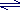# Sulfuric Acid : Production, Properties and Uses

## Properties of Sulfuric Acid (H2SO4)

• Sulfuric acid is a diprotic acid
(can donate 2 protons to a base)
• Sulfuric acid ionises (dissociates) in water in two stages:

(i)   H2SO4(l) + H2O(l) → HSO4-(aq) + H3O+(aq)

(ii)   HSO4-(aq) + H2O(l)SO42-(aq) + H3O+(aq)

• Sulfuric acid is a strong acid
(complete dissociation in water, Ka approaches infinity)
• Sulfuric acid reactions:

sulfuric acid + metal → metal sulfate + hydrogen gas

sulfuric acid + carbonate → metal sulfate + carbon dioxide gas + water

sulfuric acid + base → salt + water

sulfuric acid + ammonia → ammonium sulfate

• Sulfuric acid can take part in redox reactions.

No ads = no money for us = no free stuff for you!

## Uses of Sulfuric Acid (H2SO4)

• Sulfuric acid is the electrolyte used in lead-acid batteries (accumulators)
• Sulfuric acid is important in the production of fertilizers such as ammonium sulfate (sulfate of ammonia), (NH4)2SO4, and superphosphate, Ca(H2PO4)2, which is formed when rock phosphate is treated with sulfuric acid.
• Sulfuric acid is used to remove oxides from iron and steel before galvanising or electroplating
• Concentrated sulfuric acid (18 M) is used as a dehydrating agent, that is, to remove water, since it has a tendency to form hydrates such as H2SO4.H2O, H2SO4.2H2O, etc.

Sulfuric acid is often used to dry neutral and acidic gases such as N2, O2, CO2 and SO2

Sulfuric acid will "suck" water out of carbohydrates and some other organic compounds which contain oxygen and hydrogen. For example, sulfuric acid will "suck" water out of sucrose, C12H22O11(s), (cane sugar) to produce a spongy mass of carbon:

C12H22O11(s) + 11H2SO4 → 12C(s) + 11H2SO4.H2O

• Sulfuric acid is used in the production of nitroglycerine, an inorganic ester and organic nitrate, which is used as an explosive but can also be used as a vasodilator, a substance that dilates blood vessels and can be used in the treatment of certain types of heart disease:

 CH2ONO2 | CHONO2 | CH2ONO2

Do you know this?

Play the game now!

## Manufacture of Sulfuric Acid (H2SO4)

Most of the sulfuric acid manufactured is produced using the Contact Process.

 Combustion Chamber(combustion of sulfur) → Converter(conversion of sulfur dioxide) → Absorption Tower(sulfur trioxide absorbed into the sulfuric acid mist → Hydration of Oleumto produce sulfuric acid

The Contact Process is a process involving the catalytic oxidation of sulfur dioxide, SO2, to sulfur trioxide, SO3.

1. Solid sulfur, S(s), is burned in air to form sulfur dioxide gas, SO2
S(s) + O2(g) → SO2(g)
2. The gases are mixed with more air then cleaned by electrostatic precipitation to remove any particulate matter
3. The mixture of sulfur dioxide and air is heated to 450oC and subjected to a pressure of 101.3 - 202.6 kPa (1 -2 atmospheres) in the presence of a vanadium catalyst (vanadium (V) oxide) to produce sulfur trioxide, SO3(g), with a yield of 98%.
2SO2(g) + O2(g) → 2SO3(g)
4. Any unreacted gases from the above reaction are recylced back into the above reaction

5. Sulfur trioxide, SO3(g) is dissolved in 98% (18 M) sulfuric acid, H2SO4, to produce disulfuric acid or pyrosulfuric acid, also known as fuming sulfuric acid or oleum, H2S2O7.
SO3(g) + H2SO4 → H2S2O7

This is done because when water is added directly to sulfur trioxide to produce sulfuric acid

SO3(g) + H2O(l) → H2SO4(l)

the reaction is slow and tends to form a mist in which the particles refuse to coalesce.

6. Water is added to the disulfuric acid, H2S2O7, to produce sulfuric acid, H2SO4
H2S2O7(l) + H2O(l) → 2H2SO4(l)

The oxidation of sulfur dioxide to sulfur trioxide in step III above is an exothermic reaction (energy is released), so by Le Chatelier's Principle, higher temperatures will force the equilibrium position to shift to the left hand side of the equation favouring the production of sulfur dioxide.
Lower temperatures would favour the production of the product sulfur trioxide and result in a higher yield.
However, the rate of reaching equilibrium at the lower temperatures is extremely low.
A higher temperature means equilibrium is established more rapidly but the yield of sulfur trioxide is lower.
A temperature of 450oC is a compromise whereby a faster reaction rate results in a slightly lower yield.

Similarly, at higher pressures, the equilibrium position shifts to the side of the equation in which there are the least numbers of gaseous molecules.

2SO2(g) + O2(g) → 2SO3

On the left hand side of the reaction there are 3 moles of gaseous reactants, and the right hand side there are 2 moles of gaseous products, so higher pressure favours the right hand side, by Le Chatelier's Principle.
Higher pressure results in a higher yield of sulfur trioxide.

A vanadium catalyst (vanadium(V) oxide) is also used in this reaction in order to speed up the rate of the reaction.

Do you understand this?

Take the test now!